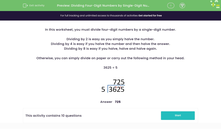# Divide Four-Digit Numbers by Single-Digit Numbers

In this worksheet, students will divide a four-digit number by a single-digit number.Key stage:  KS 3

Curriculum topic:   Number

Curriculum subtopic:   Use Four Operations for All Numbers

Popular topics:   Multiplication worksheets, Division worksheets

Difficulty level:#### Worksheet Overview

In this activity, we will be dividing four-digit numbers by a single-digit number.

To divide by 2 we halve the number.

To divide by 4 we halve the number and then halve the answer.

To divide by 8 we halve, halve and halve again!

We need to know how to divide on paper using our bus-stop method.

3625 ÷ 5Let's have a go at some questions and see how we get on.### What is EdPlace?

We're your National Curriculum aligned online education content provider helping each child succeed in English, maths and science from year 1 to GCSE. With an EdPlace account you’ll be able to track and measure progress, helping each child achieve their best. We build confidence and attainment by personalising each child’s learning at a level that suits them.

Get started